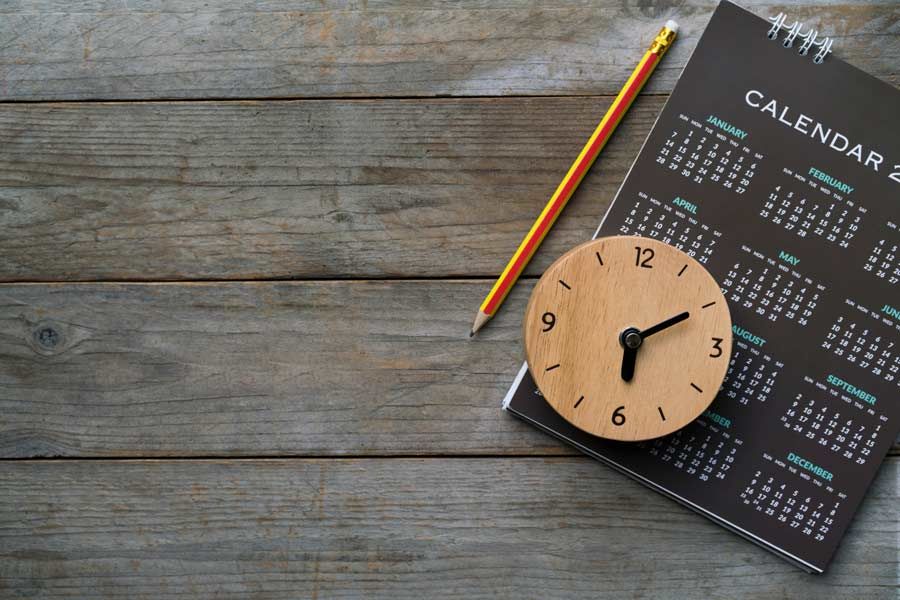# Days & Time Between Dates

Include all days?
Include end day?
Use this calculator to work out the number of hours, days and weeks between two dates of your choice. You can include and exclude specific days of the week as you want to.

Disclaimer: Whilst every effort has been made in building our calculator tools, we are not to be held liable for any damages or monetary losses arising out of or in connection with their use. Full disclaimer.

## Calculating days between two dates

If you want to know how many hours, days or weeks there are between one date and another, our online calculator is here to help you.

Perhaps you want to find out exactly how old you are, or work out how many days there are until your next birthday. Or, maybe you're looking to calculate the number of hours or days until you retire. Whatever the reason, you can use this calculator to assist you.

## How to calculate weeks between dates

To calculate the number of weeks between two dates, count the number of days between the dates and then divide your figure by 7.

This gives you a total number of weeks including a decimal. To work out the decimal as a number of days, try the following steps:

1. Work out the number of days between your start date and end date
2. Divide the number of days by 7 to get the number of weeks, with a decimal
3. Separate the figures after the decimal point & convert them to days by multiplying by 7
4. You now have your answer in whole weeks plus remainder days (part week)

### Example calculation for weeks between dates

Let's say you want to know how many weeks there are between 5th March and 21st June. We've counted the days and know that there are 108 days between these two dates. Our process to calculate the number of weeks can therefore look like this:

1. Divide 108 by 7 to get 15.42857. That means 15 whole weeks plus 0.42857 of a week
2. Multiply 0.42857 by 7 to get the number of remainder days. 0.42857 × 7 = 3 days
3. We have our answer. 15 weeks and 3 days. We can check this using our calculator.

## How many days since...?

Our date calculator allows you to look to the past. You may wish to know how many days have elapsed since you were born, since you got married or since a particular date in history (the end of World War II, the birth of Shakespeare?). To calculate the number of days that have passed, simply enter the year, month and day of the historical date in the first entry on the calculator.

If you want to look ahead in time, we've got you covered with individual pages telling you the date 30 days from today, as well as 45 days and 90 days.

### Calculating time manually

You can, of course, calculate the days or time between two dates manually, and should you wish to go down that route you'll need to remember how many days there are in each month. We know that there's 365 days in a regular year. If there's a leap year involved then an extra day is added. A leap year occurs every 4 years and means that February has 29 days instead of 28.

Then we get into months and the mnemonic to help remember the number of days in each:

"Thirty days have September, April, June, and November. February has 28 alone (or 29 in a leap year), And all the rest have 31."

So, calculating the number of days between two given dates isn't the easiest task because of the way in which our Gregorian calendar works (we've been using the Gregorian calendar for over 436 years, but many people question why we still use it).

The days between dates calculator on this page works by getting the time stamp of the start date and deducting it from the end date (or vice-versa if the end date is in the past) before calculating the number of hours, days and years between them. You can add weeks and months to a date by using our date calculator## How many days since I was born?

To calculate the number of days since you were born, simply enter your date of birth as the start date and then ensure that the end date is set to today's date (if it isn't already set, click the 'today' link that appears to the right of the box). Then click the 'Calculate' button. Alternatively, you could give my age calculator a try.

As well as telling you how many days you've been on our planet, my calculator is also able to work out what day you were born on. As an alternative, you could try the birthday calculator, which will show you all sorts of fun facts about your birthday, including the day on which you were born.

In a world where privacy of personal data is hugely important, please rest assured that I do not collect or store any of the date information you enter into this calculator.

Calculator by Alastair Hazell